# MAX2205—Detecting High Peak-to-Average Ratio Signals

### Abstract

This application note shows measurements taken using the MAX2205 RF power detector for various peak-to-average ratio signals. It also mathematically explores the theory of operation of the MAX2205.

The MAX2205 power detector's input stage is essentially two transistors arranged as shown in Figure 1. The output voltage is proportional to the input signal's voltage amplitude.Figure 1. Diagram of the MAX2205 input stage.

For complex modulations where peak-to-average ratio (PAR) varies depending on the modulation type, the MAX2205's output does not imply the exact average power. An in-depth mathematical analysis can be found in the Appendix of this application note but, basically, some correction must be applied in these circumstances. What follows is the result of an empirical study of the MAX2205 power detector's behavior with different PAR signals.

### Measurements

Measurements were made using the MAX2205EVkit (see Figure 2).Figure 2. Schematic of MAX2205EVKit.

• Signal Frequencies
• 1.9GHz
• 800MHz
• 450MHz
• Modulation Types Measured
• QPSK Modulated, 3.5dB PAR
• QPSK Modulated, 6.5dB PAR
• QAM Modulated, 6dB PAR

### Measurement Results

The plots in Figures 3 through 5 use 3.5dB PAR as a baseline or 'zero' error. R2 is adjusted to suit the frequency band and to result in a desired output-voltage range.

Figure 3. Error measurement for a signal frequency (fIN) of 1.9GHz, where:
VCC = 2.8VDC
R2 = 150Ω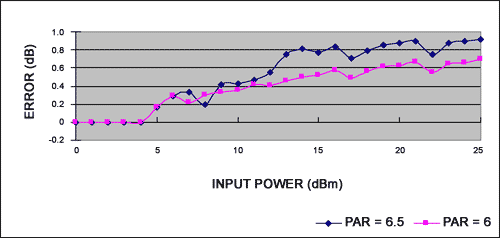Figure 3a. Error relative to signal at +25°C.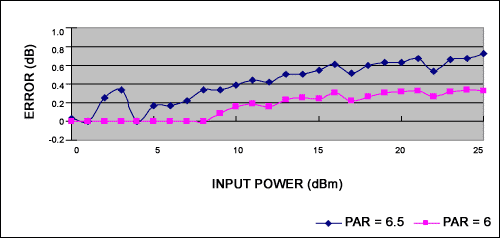Figure 3b. Error relative to signal at -40°C.Figure 3c. Error relative to signal at +85°C.

Figure 4. Error Measurement for an fIN of 800MHz, where:
VCC = 2.8VDC
R2 = 150Ω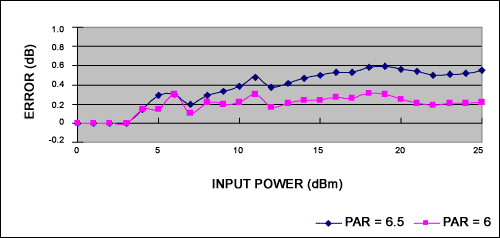Figure 4a. Error relative to signal at +25°C.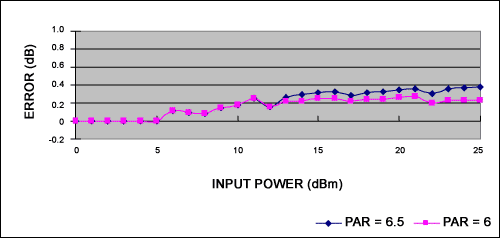Figure 4b. Error relative to signal at -40°C.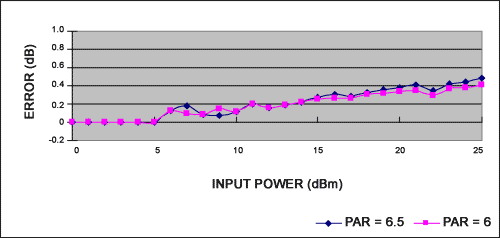Figure 4c. Error relative to signal at +85°C.

Figure 5. Error measurement for an fIN of 450MHz, where:
VCC = 2.8VDC
R2 = 330ΩFigure 5a. Error relative to signal at +25°C.Figure 5b. Error relative to signal at -40°C.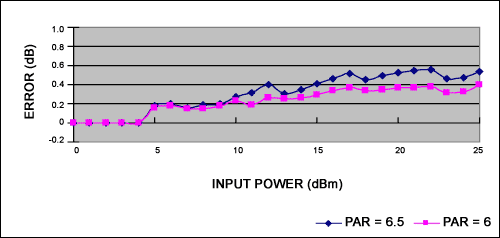Figure 5c. Error relative to signal at +85°C.

### Conclusions

1. The MAX2205 responds to the input voltage, not the square of the input voltage. When the PAR changes, the output voltage will change.
2. For higher PAR values, the resultant error is greater. At room temperature, the error for a 6.5dB PAR signal is 0.9dB at 1.9GHz, 0.55dB at 800MHz, and 0.56dB at 450MHz. Using lower coupled power (i.e., lower incident power to the detector) reduces the error, but also compresses the power detector's dynamic range. For some cases, this error is deemed acceptable and a single lookup table can be used for crest factors from 3.5dB to 6.5dB. The Appendix shows an analysis explaining why the error is reduced at a lower input power.
3. Errors are not significantly temperature dependant.
4. Multiband applications may require more than one lookup table. However, the output-voltage curves are similar over frequency, and a correction factor may be devised to allow use of only one lookup table.

### Appendix—Detailed Mathematical Analysis of a Power Detector Using Diode I/V Characteristics and a Typical Circuit

For this analysis, diode I/V characteristics are:We will perform the I/V analysis for large- and small-signal input conditions as follows.

The Figure 6 power-detector circuit has symmetric transistors Q1, Q2; I1, I2; and R1, R2. Bipolar transistor Q1 rectifies the input voltage VI. Transistor Q2 offers a DC offset voltage to balance the VO to zero when the AC input signal VAC is zero. C1 is a hold capacitor whose value can be set by the allowable voltage drop requirement of VO. The DC bias of Q1 and Q2 should be equal so as to cancel any temperature drift.Figure 6. Typical power-detector circuit.

The emitter current of Q1 is:where VQ is the bias voltage at the base of Q1, VC1 is the voltage at C1, and signal Vi = VAC x cos(ωt) is applied to Q1.

Compared to equation 1, an approximation is made based on Vi = VQ + VAC x cos(ω x t) >> VT:where:
VAC = peak amplitude of AC input signal
VQ = DC voltage difference of base and emitter
b = VAC/VT
In(b) = modified Bessel function of order n

The DC component of IE is:For conditions where VAC >> VT, I0(b) can be approximated as:Therefore,Because I = I1 constant-current regulation is in series with the emitter of a bipolar transistor, IE_DC should be equal to I1. Therefore,At the same time, considering the bipolar transistor Q2, which is identical with Q1: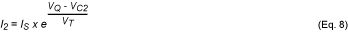where VC2 is the average DC at C2 (emitter of Q2).

For the symmetric circuit design, I1 = I2. Therefore,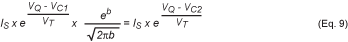From equation 9, we can derive: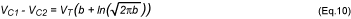We know that VO = VC1 - VC2 and b = VAC / VT. Therefore,This is the approximate relationship between the input-signal voltage and output voltage for the case where the input signal is larger.

Equation 11 shows us that:

1. VO is approximately linear in response to VAC, because VAC is included in the second term of equation 11 under a root and logarithm. This is why the output voltage varies with PAR for large input signals.
2. The temperature dependency is small for VAC >> VT.
For conditions where the input signal is small, the I0(b) in equation 4 can be approximated as:Similar to equation 9, we know that:Therefore,Using ln(1 + x)x when x is smaller gives: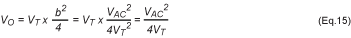Equation 15 implies that:

1. The output voltage is proportional to the square of RF input-signal voltage amplitude. The square of the voltage's amplitude is proportional to power; therefore, in the square-law region, the power detector's output voltage is proportional to the input signal's power.
2. The output voltage is inversely proportional to temperature.